• 用C++求图的最小生成树、单元点最短路径、两点之间最短路径
• 两点之间最短距离 这是我的一个测试，也是我学习html的起点，他们说一个合格的程序员必须学会html，我比他们起步晚了一些，可是我认为还来的及，以后我就用html来记录我的学习记录了。 问题的提出...
    两点之间最短距离

这是我的一个测试，也是我学习html的/起点，他们说一个合格的程序员必须学会html，我比他们起步晚了一些，可是我认为还来的及，以后我就用html来记录我的学习记录了。

问题的提出：
在二维平面的n个点上，找出其中的一对点，使得在n个点组成的所有的点中，该点对的距离最小。

一维：最接近点对问题
方法一：（暴力法）
直接使用双层遍历来计算每一对点的距离，另外新建一个变量记录两点之间距离最小的值，但是如此一来时间复杂度就变为O(n2),

方法二：(分治法）
分治法的思路:

划分成子规模点集S1和S2；
找到S1和S2的最小距离d；
合并S1和S2：利用d在S1的(m-d,m]和S2(m,m+d]中找到最大点和最小点，即p3和q3.选出最小距离，完成合并。

m如何选择
m=(max(s)+min(s))/2;

代码：talking is cheap,show me the code;
/*根据算法随便写的，返回的是两个点之间最小的距离，不是两个点的坐标，如果有问题，欢迎指正*/
/*这里的坐标默认为正序的*/
#include<iostream>
#include<vector>
using namespace std;
int search(vector<int> target)
{
int n=target.size();
if(n<2)   //如果分到一个元素就返回最大数值
return INT_MAX;
int mid=n/2;   //中线
vector<int> temp_left;
vector<int> temp_right;   temp_left.insert(temp_left.begin(),target.begin(),target.begin()+mid); //中线分割  temp_right.insert(temp_right.begin(),target.begin()+mid,target.end());
int p=search(temp_left);
int q=search(temp_right);
int d=temp_right.front()-temp_left.back();
cout<<temp_right.front()<<" "<<temp_left.back()<<endl;
return d>min(p,q)?min(p,q):d;}//返回两边和贯穿中间的最小的两点距离
int main(){
int a={-9,-6,0,4,7,9};
vector<int> target(a,a+6);
cout<<search(target);
return 0;
}

二维最接近点的问题：
思路与一维的最接近点对相似。

选取中垂线l：x=m来作为分割直线。
递归的在S1和S2找出其最小距离d1和d2，并设d=min{d1,d2}，S中最接近点对或是d，或者是某个{p，q}
合并起来

// 随便写思路的如果有误，请指正（注意返回的是两点之间的距离，不是坐标）
#include <iostream>
#include <math.h>
#include<algorithm>
using namespace std;
struct point
{
int x, y;
};
double distance(struct point p1, struct point p2)
{
return sqrt(pow(p1.x - p2.x, 2) + pow(p1.y - p2.y,2));
}
double search(struct point p[],int pl,int pr)
{
if (pl == pr)
return INT_MAX;
int mid = (pl + pr) / 2;
double d_left = search(p, pl, mid);
double d_right = search(p, mid + 1, pr);
double temp_d = min(d_left, d_right);
while (abs(p[pl].y - p[mid].y) > temp_d&&pl < pr)//如果仅仅是单边距离就已经大于上一次比较返回的d就没必要在接下来的敌方继续比较了。
pl++;
while (abs(p[pr].y - p[mid].y) > temp_d&&pl < pr)
pr--;
for (int i = pl; i < pr; i++)
{
for (int j = i + 1; j < pr; j++)
{
if (temp_d < abs(p[i].x - p[j].x))//同理与上面的注释
break;
else
temp_d = min(temp_d, distance(p[i], p[j]));//找到最小的距离
}
}
return temp_d;
}
int main()
{
struct point p;
int n;
cin >> n;
for (int i = 0; i < n; i++)
{
cin >> p[i].x;
cin >> p[i].y;
}
cout<<search(p, 0, n - 1);
}




展开全文• 一点有关任意两点最短路径的思考！！仅仅是个人拙见。
• Dijkstra算法是求源点到其它顶点的最短路径。怎样求任意个顶点之间最短路径？
• 两点之间最短路径的计算-floyd算法 Floyd算法的核心内容 算法过程图解 伪代码 完整代码 void floyd() { for (int k = 0; k < n; ++k) { for (int i = 0; i < n; ++i) { for (int j = 0; j < n; +...
两点之间最短路径的计算-floyd算法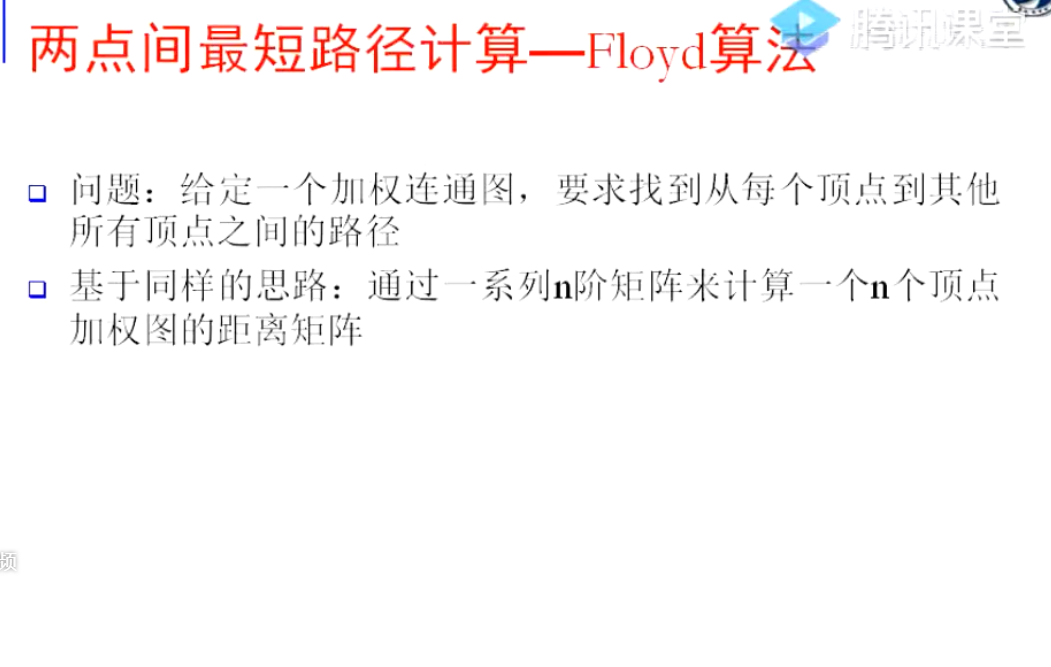Floyd算法的核心内容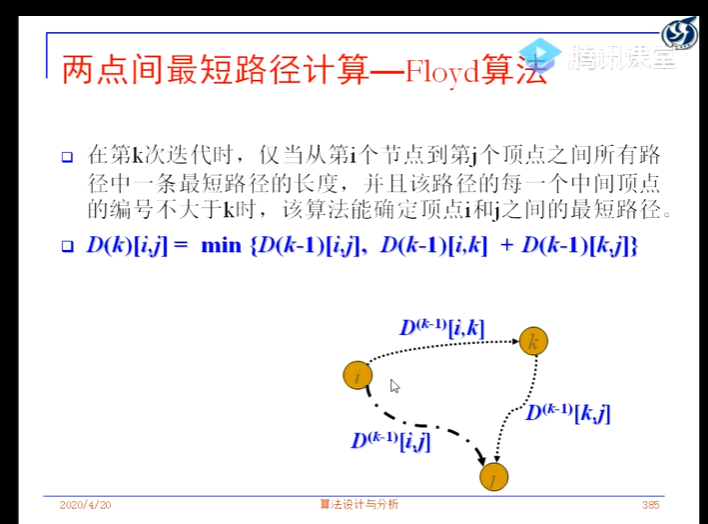算法过程图解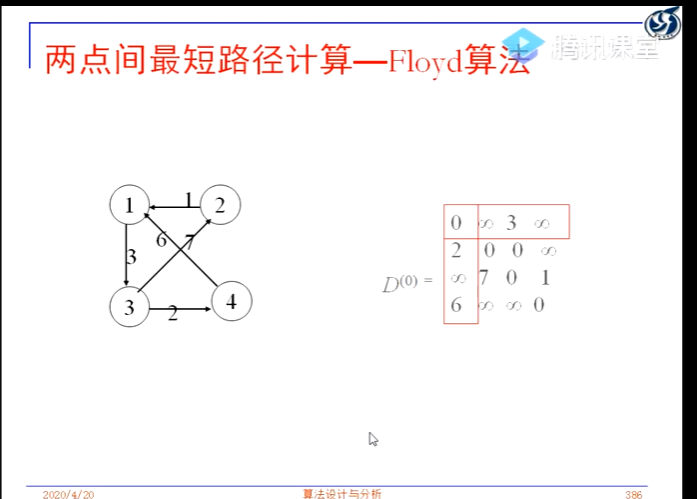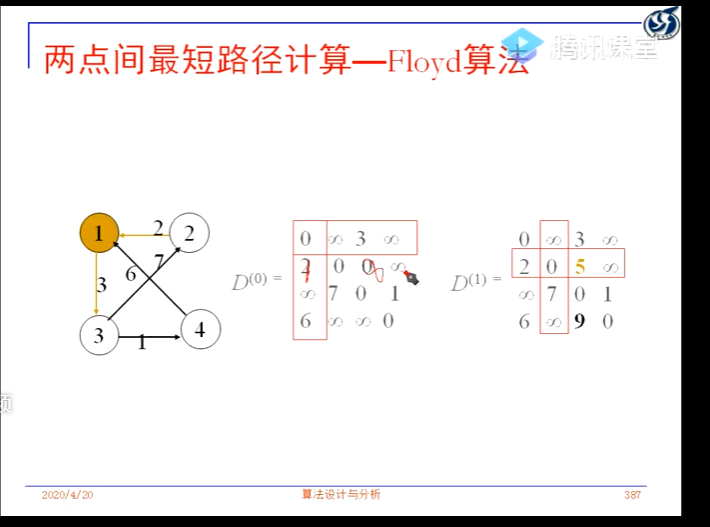伪代码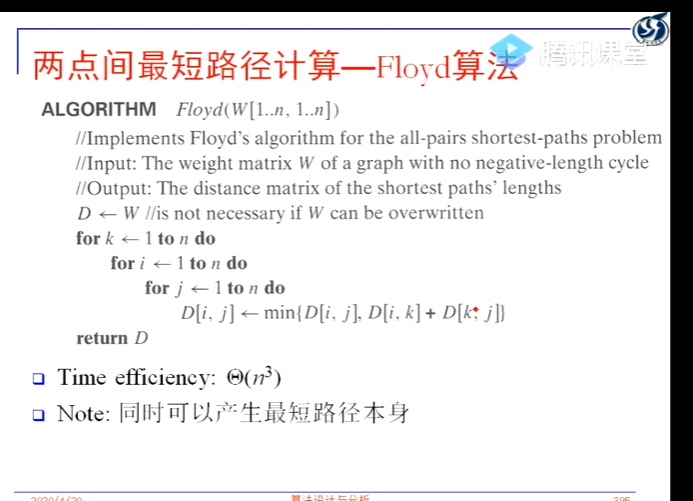完整代码
void floyd()
{
for (int k = 0; k < n; ++k)
{
for (int i = 0; i < n; ++i)
{
for (int j = 0; j < n; ++j)
{
//在当前i到j经过k点的路径与直连的路径中选最短
matrix[i][j] = min(matrix[i][j], matrix[i][k] + matrix[k][j]);
}
}
}
}



展开全文• 通过输入，能够实现找到最短路径。源代码能运行，简单易懂
• 1 先求出原点离中间之间最短路径，然后，基于已经求出的最短路径，进一步求出它们之间最短路径。 转载于:https://www.cnblogs.com/wwwfj/p/3350166.html
1 先求出原点离中间之间的最短路径，然后，基于已经求出的最短路径，进一步求出它们之间的最短路径。
转载于:https://www.cnblogs.com/wwwfj/p/3350166.html
展开全文• /* *求有向网中的任意两点的最短路径 *弗洛伊德算法的核心是 运用一个path二维数组 和一个A...*运用三层循环 （算法的核心） 计算任意两点之间最短路径长度 将经过的节点的下标存储在path数组中去 *递增的思想 *创...
/*
*求有向网中的任意两点的最短路径
*弗洛伊德算法的核心是  运用一个path二维数组  和一个A二维数组
*path数组用来保存中间路径经过的节点
*A数组用来保存任意两个顶点之间的最短路径长度
*最后输出A数组运用了递归输出的思想
*先初始化path数组和A数组
*运用三层循环  （算法的核心） 计算任意两点之间的最短路径长度  将经过的节点的下标存储在path数组中去
*递增的思想
*创建一个有向网   输出一个临接矩阵
*/
#include<stdio.h>
#include<stdlib.h>
#include<string.h>
#define  OK 1
#define ERROR 0
#define   VERTEXNUM 100//最大顶点数
#define  NAME_SIZE 255//字符串的最大长度
#define MAX_INT 32726 //权重最大值
typedef int Statu;//返回值的数据类型
typedef  char*VertexType;//字符串的数据类型
typedef int ArcType;//权值数组的数据类型
typedef struct  amgraph
{
VertexType vexs[VERTEXNUM];//图的顶点数组
ArcType arcs[VERTEXNUM][VERTEXNUM];//图的权值的二维数组
int n;//图的顶点数
int e;//图的边数
}AMGraph;//图的结构体
int  path[VERTEXNUM][VERTEXNUM];//用于保存进过的中间路径的节点的下标
int A[VERTEXNUM][VERTEXNUM];//经过的路径节点的权值
void main()
{
test();//测试函数

}
void test();//测试函数
Statu create_DN(AMGraph*G);//创建有向网
int Locate_vex(AMGraph*G,VertexType vex);//定位函数
void Floyed_function(AMGraph*G);//弗洛伊德算法
void Dispath(int A[][VERTEXNUM],int path[][VERTEXNUM],int n);
//n代表当前图的顶点数
//最短路径第一输出函数  主要用于输出权值
void Ppath(int path[][VERTEXNUM],int i,int j);//用于输出路径节点的下标
//创建一个有向网
Statu create_DN(AMGraph*G)//创建有向网
{
int i,j;//循环变量
VertexType  vex1,vex2;//字符串的临时变量
int x,y;//定位函数的临时变量
int now_weight;//当前的权重
printf("请输入有向网的顶点数：");
scanf("%d",&G->n);
printf("请输入有向网的边数：");
scanf("%d",&G->e);
//初始化顶点的信息
for(i=0;i<G->n;i++)
{
G->vexs[i]=(VertexType)malloc(sizeof(char)*10);//动态申请存储空间
printf("顶点%d：",i+1);
scanf("%s",G->vexs[i]);

}
//初始化权值数组
for(i=0;i<VERTEXNUM;i++)
for(j=0;j<VERTEXNUM;j++)
{
G->arcs[i][j]=MAX_INT;//权值初始化

}
//初始化边的信息
for(i=0;i<G->e;i++)
{
vex1=(VertexType)malloc(sizeof(char)*10);//动态申请存储空间
vex2=(VertexType)malloc(sizeof(char)*10);//动态申请存储空间
printf("顶点：");
scanf("%s",vex1);
printf("邻接点：");
scanf("%s",vex2);
printf("请输入当前的权重：");
scanf("%d",&now_weight);
x=Locate_vex(G,vex1);
y=Locate_vex(G,vex2);
if(x==-1||y==-1)
{
return ERROR;
}
else
{
G->arcs[x][y]=now_weight;//创建的是有向网
}

}
return OK;
}
int Locate_vex(AMGraph*G,VertexType vex)
{
int index=0;
while(index<G->n)
{
if(strcmp(vex,G->vexs[index])==0)
{
break;
}
else
{
index++;
}
}
return (index==G->n ? -1 : index);
}
void Floyed_function(AMGraph*G)//弗洛伊德算法
{
int i,j;//循环变量
int k;
for(i=0;i<G->n;i++)
for(j=0;j<G->n;j++)
{
path[i][j]=-1;//path数组初始化
A[i][j]=G->arcs[i][j];
//初始化A数组  有边的存在 存储权值 没有边的存在 即为无穷大
}
//算法的核心  三层循环
for(k=0;k<G->n;k++)
for(i=0;i<G->n;i++)
for(j=0;j<G->n;j++)
if(A[i][j]>A[i][k]+A[k][j])
{
A[i][j]=A[i][k]+A[k][j];
path[i][j]=k;//存储路径

}

Dispath(A,path,G->n);//输出任意两个邻接点的最短路径长度

}
void Ppath(int path[][VERTEXNUM],int i,int j)//输出最短路径经过的中间节点
{
int k;
k=path[i][j];
if(k==-1)//两个顶点之间无中间节点
{
printf("%d->",i);
printf("%d",j);
}
else{
Ppath(path,i,k);//递归的思想   输出最短路径经过的节点
printf("%d->",k);
Ppath(path,k,j);}

}
void Dispath(int A[][VERTEXNUM],int path[][VERTEXNUM],int n)//依次输出最短路径的权值
{
int i,j;//循环变量
for(i=0;i<n;i++)
for(j=0;j<n;j++)
{
if(A[i][j]==MAX_INT)

if(i!=j)//排除顶点本身的情况
{
printf("从%d到%d没有路径\n",i,j);

}
else
{
printf("%d到%d的最短路径长度为\n",i,j);
// printf("path：");
printf("%d->",i);
Ppath(path,i,j);
printf("%d",j);
printf("路径长度为：%d\n",A[i][j]);
}

}

}

void test()
{
int i,j;//循环变量
AMGraph*G;
G=(AMGraph*)malloc(sizeof(AMGraph));
int result=create_DN(G);
if(result==ERROR)
{
printf("有向网创建失败！\n");
return ;
}
else//输出邻接矩阵
{
printf("该有向网的邻接矩阵为：\n");
printf("\t");
//输出第一行的顶点
for(i=0;i<G->n;i++)
{
printf("\t%s",G->vexs[i]);
}
printf("\n");//换行输出
//输出边的信息
for(i=0;i<G->n;i++)
{
printf("\t%s",G->vexs[i]);
for(j=0;j<G->n;j++)
{
if(G->arcs[i][j]==MAX_INT)
printf("\t∞");
else
printf("\t%d",G->arcs[i][j]);

}
printf("\n");//换行

}
}
printf("\n");
printf("最短路径及其经过的节点为:\n");
Floyed_function(G);

}



展开全文C语言数据结构
• 主要特点是以起始为中心向外层层扩展，直到扩展到终点为止。Dijkstra算法是很有代表性的最短路径算法，在很多专业课程中都作为基本内容有详细的介绍，如数据结构，图论，运筹学等等。注意该算法要求图中不存在负权...
• MAX——定义两点之间若无路径赋予的最大值 变量： DIST[N]——存储已经搜寻到的最短路径 Is[N]——存储节点是否被遍历的状态 Path[N]——图之间的路径矩阵 Road[N]——存储最短路径时该节点的上一节点 算法...Dijkstra算法
• 本文主要讲述最短路径算法，一个主要原因是网上的“基于Matlab实现的两点之间最短路径算法”存在各种实现错误，目前为止还没有找到一个完全正确的。所以，本人改正相关错误，上传个正确的版本，即：采用Matlab实现的...
• 给定一个乡镇网络图，求取个乡镇的最短路径，并输出路径，以及路径距离的大小。
• // 保存start到其他各点最短路径的字符串表示 for (int i = 0; i ; i++){ path[i] = new String(point[start] + pathTip + point[i]); } int[] visited = new int[n]; // 标记当前该顶点的最短路径...
• 两点顶点之间最短路径问题 基于matlab的dijkstra算法 前言 看了很多篇介绍dijkstra算法的博客，没发现对于程序来说很多的地方都有问题。 一、函数代码 代码如下（示例）： function [ mydistance,mypath] = ...dijkstra 算法
• 防止相异的两点距离为0的情况出现  array[j][i]  =  array[i][j];  }  array[i][i]  =   0 ;  }   return  array;  }   // 注意：k 的循环要在最外层   static   int [][] shortestpath() ... {   ...string class 测试
• using System; using System.Collections.Generic; using System.Linq; using System.Text;...//迪杰斯特拉算法 计算两点最短距离 namespace Dijkstra { class Program { /// /// 邻接矩阵 ///
• 我对弗洛伊德算法的理解(求图中任意两点之间最短距离) 基本过程： for k = 1:n for i = 1:n for j = 1:n if route(i,k)+route(k,j) (i,j) route(i,j) = route(i,k) + route(k,j);
• ## 两点之间弧线最短

千次阅读 2013-11-28 23:51:23
两点之间弧线最短  道理是直的，路永远是弯的 只会走直路是很难达到目的的，因为自然本来就是弯弯曲曲的。   我们中国的大江大河都是由西发源，然后向东流，但没有一条是笔直入海的，因为那样...
• Dijkstra算法实现两点之间最短路径算法[VC++
• 无向图两点之间最短距离怎么求？有什么算法可以实现啊。头疼死了，好几天了，求指点，给个具体点的代码。c语言
• 两点之间最短路径（Floyd算法）源代码 调试通过的
• 上面已经给出了两点之间的线路路径，不能再通过两点时间的路径来计算他们最短路径了，根据以往的经验要想让两点之间的路程变短，只能引入第三个点，通过第三个点的中转才能缩短两点之间的路径。 通过上图可知由4-&.....
• 主要为大家详细介绍了python广度优先搜索得到两点最短路径，具有一定的参考价值，感兴趣的小伙伴们可以参考一下
• 求从P经过A、B、C、D、E、F、G、H、I后到达F，不必考虑这9个的相对顺序，求P到J的最短路径，
• 最短路径算法1.Dijkstra算法2.Bellman-Ford算法3.SPFA算法4.Floyd算法几种最短路径算法的对比Dijkstra算法、Bellman-Ford算法和SPFA算法的对比Dijkstra算法和Floyd算法的...多源最短路算法：求任意两点之间最短路径.算法
• 主要为大家详细介绍了JS实现深度优先搜索求解两点最短路径，具有一定的参考价值，感兴趣的小伙伴们可以参考一下
• Finding the shortest path between two points on a graph is a common problem in data structures, ... 在数据结构中，尤其是在处理优化时，通常要在图形上找到两点之间最短路径。 A graph is a series of...算法 python java 人工智能 大数据
• 实验三：C#实现求图的任意两点最短路径及距离 实验目的 定义Graph类、Node类，从文件中读取图结构；给定两个顶点，给出两个顶点的最短路径（如果没有连通，请给出）。把图进行可视化展示（一个适当大小的图），...C# Floyd算法
• 在计算两点之间最短路径时，如果两点之间存在其他的点，那么可以将最短路径的情况分为两类，经过某个点和不经过这个点。那么在求有向图中ab两点的最短路径时，遍历剩下的点，比较在a到b的路径中是经过Vi距离短...算法
• 我们之前做的dijkstra算法只能实现两点之间的1条最短路径的计算。dijkstra算法需要和yen算法结合，才能实现获取两点之间的k条最短路径。关于后面的数据准备有疑惑的，可以参考上篇博文WebGIS开发之最短路径分析入门 ...dijkstra postgis...